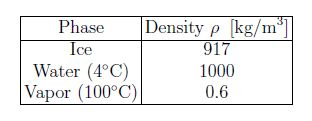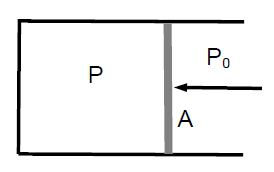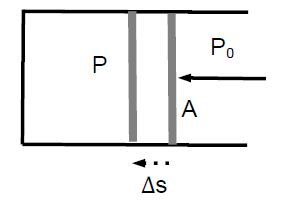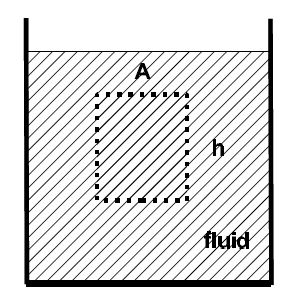Physics

# Fluids at Rest: Mathematical Solution

The density of an object is defined as the ratio of mass in divided by its volume,

P = m/V

The unit of density is [p] = [m]/[V] = kg/m3. The density of liquids and solids are of the order of thousands of kilograms per cubic meter [p ≈ 103 kg/m3] and is about a thousand times smaller [p ≈ 1 kg/m3] for gases, which is consistent with the times greater distance between atoms (molecules). For H2O, the respective densities are:H2O is has many unusual properties: among them is the greater density in the liquid phase compared to the solid phase. Note that the density of vapor strongly depends on temperature and pressure [see below].

Example: The approximate density of the human body is close to that of (liquid) water p ≈ 1000 kg/m3. Find the volume of a person.

Solution: For m = 70 kg [or 155 lbs], so for the volume,

V = M/P = (70 kg / 100 kg/m3) = 7.0 x 10-2 m3 = 7.0 x 104 cm3.

This is equivalent to the volume of a cube with length a ≈ 41 cm.

The response [e.g., the deformation] of a system to an external force F also depends on the size of the system. Pressure is defined as the ratio of force divided by the area,

P = F/A

The SI unit of pressure is [P] = [F]/[,11] = N/rn2 = Pa [“Pascal”]. Normal atmospheric pressure is Patm = 1.015 x 105 Pa. Pressure explains why we sleep in beds rather than on the floor. the forces exerted by the mattress and the floor on the person are equal [and equal to the weight of the person]. A mattress adjusts its shape to the person’s body, thereby increasing the area and decreasing the pressure.The pressure inside and outside a piston is P and P0 < P, respectively. If the cross-sectional area of the piston is A, a force F = (P – P0) • A must be applied to move the piston to the left.If the piston is displaced by Δs, work is done on the gas: W=F•As. We find W = F Δs. We find W = PA*Δs) = P(A*Δs) = – P ΔV, where we used A Δs = -Δs [negative, because the volume of the gas decreases]. This yields an alternative definition of pressure

P = – (W / ΔV)

which is useful in many applications, especially when the change in the volume ΔV is small and the change in pressure can be ignored.We examine the effect of gravity on a fluid. We consider a fluid element with a cross-sectional area A and a height h. The forces on the element are from the top Ft, the bottom Fb, the left Fl, and the right Fr.There is no difference between left and right [since we cannot differentiate between the volume element and its mirror image] so that Fl = Fr. Since the volume element is in mechanical equilibrium, the difference between the force from the bottom and top is equal to the weight: Fb – Ft = mg. This difference is produced by the pressure difference Fb — Ft = (Pb — Pt)A. The mass of the element is m = pV = pAh . We get (Pb — Pt)A = pAhg , or (P — Pt) = pgh . If P0 is the pressure on top of the surface, the pressure at the distance h below the surface is:

P = P0 + pgh.

That is, the pressure increases with the depth below the surface of fluid at rest: hydrostatic pressure.